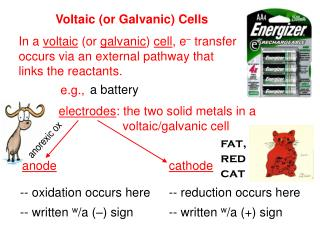Download Presentationanorexic ox

# anorexic ox - PowerPoint PPT PresentationDownload Presentation## anorexic ox

- - - - - - - - - - - - - - - - - - - - - - - - - - - E N D - - - - - - - - - - - - - - - - - - - - - - - - - - -
##### Presentation Transcript

1. anode cathode Voltaic (or Galvanic) Cells In a voltaic (or galvanic) cell, e– transfer occurs via an external pathway that links the reactants. a battery e.g., electrodes: the two solid metals in a voltaic/galvanic cell anorexic ox fat, red cat -- oxidation occurs here -- reduction occurs here -- written w/a (–) sign -- written w/a (+) sign

2. e– e– Zn anode Cu cathode NO3– Na+ Zn2+ Cu2+ Cu2+ Zn2+ NO3– NO3– Consider a solution of Zn(NO3)2(aq) and Cu(NO3)2(aq) with electrodes as shown… salt bridge containing electrolyte (e.g., NaNO3) in a porous gel (needed to neutralize both solutions) -- Zn anode dissolves into sol’n -- Cu2+ plates out as Cu on the cathode WHY do the e– go the way they do?

3. Difference in is important; PE charge Cell EMF > PE of anode’s e– PE of cathode’s e– Thus… anode’s e–s spontaneously flow towards cathode, if given a path. # of charges is NOT. Voltage (or “potential”) difference is also called the electromotive force (emf).

4. (Look it up!) For a particular cell, (i.e., a particular anode and cathode), the cell’s emf is written Ecell and is called the cell potential. it is + for spontaneous cell reactions -- it depends on materials, conc., and temp. -- -- standard emfs occur at 25oC -- To calculate Ecell, look up tabulated standard reduction potentials for each half-cell… e.g., Ag+(aq) + e–Ag(s) Eored = +0.80 V …and then use the equation: Eocell = Eored,cath – Eored,an

5. (Eored = –0.76 V) The reference point for reduction potentials is the standard hydrogen electrode (SHE): 2 H+(aq, 1 M) + 2 e–H2(g, 1 atm) Eored = 0 V This is analogous to the arbitrary ref. line when calc. PEg in physics. J Multiples of coefficients don’t affect Eored. V = C e.g., Zn2+(aq, 1 M) + 2 e– Zn(s) 2 Zn2+(aq, 1 M) + 4 e– 2 Zn(s) Because the same chemicals are used for each, AAA-, AA-, C-, and D-batteries all have an emf of 1.5 V.

6. Eo = Cu2+ Cu Cu2+ solution Cr metal Cu metal Cr2+ solution For Cr(s) + Cu2+(aq)  Cr2+(aq) + Cu(s), Eocell is measured to be 1.25 V. Given that Eored for Cr2+ to Cr is –0.91 V, find Eored for the reduction of Cu2+ to Cu. Eocell = Eored,cath – Eored,an Eored, cath – 1.25 V = –0.91 V –0.91 V (Cu2+ Cu) +0.34 V

7. A galvanic cell has half-rxns: (a) Al3+(aq) + 3 e–Al(s) Eored = –1.66 V (b) Ba2+(aq) + 2 e– Ba(s) Eored = –2.90 V Calculate Eocell and write the balanced equation. For a galvanic cell, Eo must be > 0. Thus, (a) represents the cathode and (b) represents the anode. Eocell = Eored,cath – Eored,an Eocell = –1.66 V – –2.90 V = 1.24 V 2 3 2 3 Al3+(aq) + Ba(s) Al(s) + Ba2+(aq)